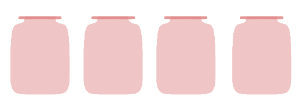Study P2 Mathematics Multiplication and Division 1 - Geniebook# Multiplication And Division 1

• Understanding Multiplication

## Topic Recap:

### Understanding Multiplication As Repeated Addition/Equal Grouping

• We multiply by putting equal groups together. Equal groups contain the same number of objects.
• To multiply, we use repeated addition or multiplication to find the total number of objects.
For example:
$$2 + 2 + 2 = 6$$ or $$2 \times 3 = 6$$
• We can write a multiplication equation in the following way:Example:Question 1:

Look at the following picture.1. There are __________ equal groups.

1. $$4$$
2. $$2$$
3. $$8$$

(1) $$4$$

1. Each group has __________ cupcakes.

1. $$4$$
2. $$2$$
3. $$8$$

(2) $$2$$

1. Which of the following best represents the picture above?

1. $$2$$ groups of $$4$$.
2. $$2$$ fours
3. $$4$$ groups of $$2$$.
4. $$​4 + 4 ​$$

(3) $$4$$ groups of $$2$$.

Question 2:

How many stars are there altogether?1. $$9$$
2. $$11$$
3. $$21$$
4. $$24$$

Solution:

\begin{align}​​ 8 + 8 + 8 &= 24 \\[2ex] 3 \times 8 &= 24 \end{align}

(4) $$24$$

Question 3:

How many bananas are there altogether?1. $$9$$
2. $$16$$
3. $$20$$
4. $$25$$

Solution:

\begin{align}​​ 5 + 5 + 5 + 5 = 20 \\[2ex] 4 \times 5 = 20 \end{align}

(3) $$20$$

Question 4:

Which of the following equations does not represent the following?1. $$5 + 5 + 5 + 5 + 5 + 5 = 30$$
2. $$6$$ fives $$= 30$$
3. $$6 \times 5 = 30$$
4. $$5 \times 5 \times 5 \times 5 \times 5 \times 5 = 30$$

(4) $$5 \times 5 \times 5 \times 5 \times 5 \times 5 = 30$$

## 1. Understanding Multiplication

To write a multiplication equation, we need to know the number of groups and the number of items in each group. We can then write the multiplication equation in the following way:To find the total number of pencils in the picture given, we multiply

$$10$$ by $$2$$. This means that a group of $$10$$ is multiplied $$2$$ times.

$$10 \times 2 = 20$$

We can also multiply $$2$$ by $$10$$

$$2 \times 10 = 20$$

$$10 \times 2$$’ and ‘$$2 \times 10$$’ have the same values.

Question 1:

Look at the picture below.1. Choose the correct option to represent the following statement:

Multiply $$6$$ by $$3$$

1. $$6 + 3$$
2. $$6 × 3$$

(2) $$6 × 3$$

1. How many sandwiches are there in the picture above?

1. $$9$$
2. $$18$$

Solution:

$$6 × 3 = 18$$

(2) $$18$$

Question 2:

Which of the multiplication equations represents the following?

Multiply $$8$$ by $$4$$.1. $$8 + 4 = 12$$
2. $$8 \times 4 = 32$$

(2) $$8 \times 4 = 32$$

Question 3:

Which of the following sets of multiplication equations best represents the following?1. $$5 \times 7 = 35$$ and $$7 \times 5 = 35$$
2. $$5 \times 5 = 35$$ and $$7 \times 5 = 35$$

Solution:

There are $$7$$ groups of $$5$$ buttons each.

(1) $$5 \times 7 = 35$$ and $$7 \times 5 = 35$$

Question 4:

How many stickers are there altogether?1. $$8$$
2. $$9$$
3. $$15$$
4. $$20$$

Solution:

$$5 × 3 = 15$$ or $$3 × 5 = 15$$

(3) $$15$$

Question 5:

How many tigers are there altogether?1. $$7$$
2. $$9$$
3. $$12$$
4. $$16$$

Solution:

$$4 \times 3 = 12$$

(3) $$12$$

Question 6:

How many doughnuts are there in all?1. $$12$$
2. $$18$$
3. $$27$$
4. $$36$$

Solution:

$$9 × 3 = 27$$ or $$3 × 9 = 27$$

(3) $$27$$

Question 7:

Look at the picture below.1. How many jars are there?
1. $$3$$
2. $$4$$
3. $$5$$

(2) $$4$$

1. Kelly puts 6 cookies in each jar. How many cookies are there altogether?1. $$10$$
2. $$16$$
3. $$18$$
4. $$24$$

Solution:

$$4 \times 6 = 24$$ or $$6 \times 4 = 24$$

(4) $$24$$

Question 8:

There are $$7$$ mangoes in each basket. How many mangoes are there altogether?1. $$10$$
2. $$18$$
3. $$21$$
4. $$28$$

Solution:

$$7 × 3 = 21$$ or $$3 × 7 = 21$$

(3) $$21$$

Question 9:

Ashley collected some bottles for recycling. She put $$9$$ bottles in each bag. How many recycled bottles did she collect in all?1. $$14$$
2. $$25$$
3. $$40$$
4. $$45$$

Solution:

$$5 × 9 = 45$$ or $$9 × 5 = 45$$

(4) $$45$$

## Challenge yourself!

Question 1:

What is the missing number in the given multiplication equation?

$$5 \;\times$$ __________ $$= 30$$1. $$5$$
2. $$6$$
3. $$7$$
4. $$8$$

Solution:

There are $$5$$ groups with $$6$$ ribbons each.

$$5 \times 6 = 30$$

So, the missing number in the equation is $$6$$.

(2) $$6$$

Question 2:

What is the value of $$\text{A}$$?

$$10 \times \text{A} = 40$$1. $$8$$
2. $$10$$
3. $$3$$
4. $$4$$

Solution:

There are $$10$$ turtles in each row. There are $$4$$ rows.

$$10 \times 4 = 40$$

So, the value of $$\text{A}$$ is $$4$$.

(4) $$4$$

• Multiplication is represented as ‘’.
• When we multiply two numbers, say $$6$$ by $$3$$, it is written as $$6 \times 3$$.
• The values of $$6 \times 3$$ and $$3 \times 6$$ are the same.

Continue Learning
Numbers To 1000 Multiplication And Division 1
Multiplication And Division 2 Addition And Subtraction 1
Addition And Subtraction 2 Fractions 1
Length 1 Mass 1
Volume 1 Money 1
Time 1 Shapes And Patterns
Picture Graphs 1 Model Drawing 1
Model Drawing 4PrimaryPrimary 1Primary 2EnglishMaths
Numbers To 1000
Multiplication And Division 1
Multiplication And Division 2
Fractions 1
Length 1
Mass 1
Volume 1
Money 1
Time 1
Shapes And Patterns
Picture Graphs 1
Model Drawing 1
Model Drawing 4Primary 3Primary 4Primary 5Primary 6SecondarySecondary 1Secondary 2Secondary 3Secondary 4